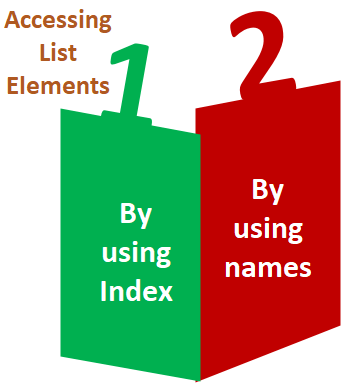# How to access the List elements by using index and name in R?

## Accessing List Elements

R provides two ways through which we can access the elements of a list. First one is the indexing method performed in the same way as a vector. In the second one, we can access the elements of a list with the help of names. It will be possible only with the named list.; we cannot access the elements of a list using names if the list is normal.Let see an example of both methods to understand how they are used in the list to access elements.

Example 1: Accessing elements using index

``````# Creating a list containing a vector, a matrix and a list.
list_data <- list(c("Shubham","Arpita","Nishka"), matrix(c(40,80,60,70,90,80), nrow = 2),
list("BCA","MCA","B.tech"))
# Accessing the first element of the list.
print(list_data)

# Accessing the third element. The third element is also a list, so all its elements will be printed.
print(list_data)
``````

Output:

``````[]
 "Shubham" "Arpita"  "Nishka"

[]
[][]
 "BCA"

[][]
 "MCA"

[][]
 "B.tech"
``````

Example 2: Accessing elements using names

``````# Creating a list containing a vector, a matrix and a list.
list_data <- list(c("Shubham","Arpita","Nishka"), matrix(c(40,80,60,70,90,80), nrow = 2),list("BCA","MCA","B.tech"))
# Giving names to the elements in the list.
names(list_data) <- c("Student", "Marks", "Course")
# Accessing the first element of the list.
print(list_data["Student"])
print(list_data\$Marks)
print(list_data)
``````

Output:

``````\$Student
 "Shubham" "Arpita"  "Nishka"

[,1] [,2] [,3]
[1,]   40   60   90
[2,]   80   70   80

\$Student
 "Shubham" "Arpita"  "Nishka"

\$Marks
[,1] [,2] [,3]
[1,]   40   60   90
[2,]   80   70   80

\$Course
\$Course[]
 "BCA"
\$Course[]
 "MCA"
\$Course[]
 "B. tech."``````